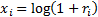/

### Extreme Events – Specimen Question A.2.1(a) – Answer/Hints

Q. Calculate the mean, (sample) standard deviation, skew and (excess) kurtosis of [Index A’s] log returns over the period covered by the above table.

The logged returns of A, i.e.are:

 Period A (logged return) 1 -0.2890163 2 0.0009995 3 0.2167230 4 0.0760347 5 -0.0140989 6 0.0049875 7 -0.0060181 8 0.0099503 9 0.0704585 10 -0.1278334 11 0.0069756 12 0.0079682 13 -0.0387408 14 0.0276152 15 -0.0439519 16 -0.0120726 17 -0.0725707 18 0.0629748 19 0.0353671 20 0.0178399

The mean, (sample) standard deviation, skew and (excess) kurtosis of A’s log returns can be calculated in a variety of ways, including:

i.         In a Microsoft Excel spreadsheet using the in-built functions AVERAGE, STDEV, SKEW and KURT respectively;

ii.       In a Microsoft Excel spreadsheet using the Nematrian web functions MnMean, MnStdev, MnSkew  and MnKurt respectively;

iii.      In VBA, the built-in programming language packaged with Microsoft Excel, using:

-          The VBA equivalents to (i), i.e. Excel.WorksheetFunction.Average, Excel.WorksheetFunction.StDev, Excel.WorksheetFunction.Skew and Excel.WorksheetFunction.Kurt respectively; or

-          The VBA equivalents to (ii), which if you have e.g. used the Nematrian website’s, automatic code generator or have loaded up a prepopulated spreadsheet from the Nematrian spreadsheet library will also be called MnMean, MnStdev, MnSkew  and MnKurt respectively;

iv.     Interactively, via the MnMean, MnStdev, MnSkew  and MnKurt webpages.

In each case the answers supplied (possibly with some rounding error) are:

 Statistic Value mean -0.0033204 stdev 0.0954161 skew -0.9253403 kurt 4.6238918